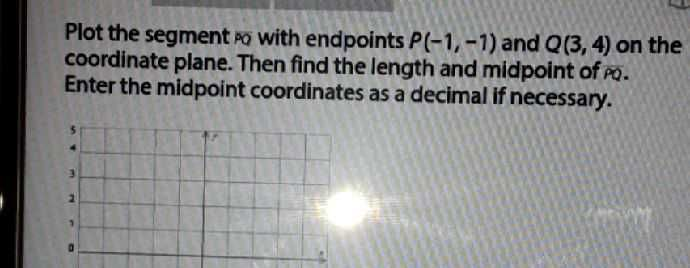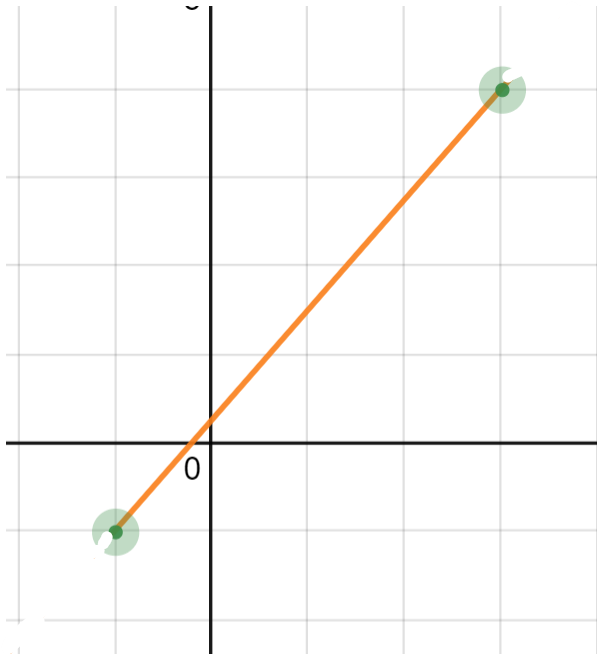### ¿Todavía tienes preguntas de matemáticas?

Pregunte a nuestros tutores expertos
Algebra
PreguntaPlot the segment $$Q Q$$ with endpoints $$P ( - 1 , - 1 )$$ and $$Q ( 3,4 )$$ on the coordinate plane. Then find the length and midpoint of $$P Q$$ . Enter the midpoint coordinates as a decimal if necessary.

(1,2.5)